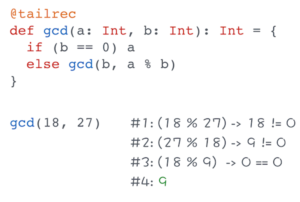Refcards Trend Reports Over 2 million developers have joined DZone. Join Today! Thanks for visiting DZone today,

Integrating PostgreSQL Databases with ANF: Join this workshop to learn how to create a PostgreSQL server using Instaclustr’s managed service

Mobile Database Essentials: Assess data needs, storage requirements, and more when leveraging databases for cloud and edge applications.

Monitoring and Observability for LLMs: Datadog and Google Cloud discuss how to achieve optimal AI model performance.

Automated Testing: The latest on architecture, TDD, and the benefits of AI and low-code tools.

# Scala: Recursive Functions

### A quick tutorial on some of the features and quirks of recursive functions in Scala, with examples of recursion and tail recursion.

· Tutorial
Save
15.56K Views

i enrolled in the functional programming in scala specialization on coursera. it consists of 5 courses which will take around 180 days. the first course in the specialization is functional programming principles in scala. i already completed the first week and learned some stuff about recursive functions. so it’s time to share some knowledge.

functions are an integral part of modern programming languages. in oop they are called methods; in a functional world their name is functions, as you may guess. i’ve already published multiple articles about scala functions . in this post, i want to speak exactly about recursive functions.

let’s start with the definition. a recursive function is a function which calls itself. that’s why it’s important to have some condition for stopping. of course, that is if you don’t want to create an infinite loop.

recursion could be applied to many common problems, which you are used to solving with the help of regular ``` loops ``` for and ``` while ``` . for example, how to calculate the sum of numbers between two numbers? we create an array of all elements and then iterate through it, adding all elements.

``````def standardsum(a: int, b: int): int = {
if (a > b) 0
else {
var result = 0
for (number <- a to b)
result += number
result
}
}

//55
standardsum(0, 10)``````

the same result can be achieved with help of recursive function:

``````def recursivesum(a: int, b: int): int = {
if (a > b) 0
else {
a + recursivesum(a+1, b)
}
}

//55
recursivesum(0, 10)``````

as you see we return expression ``` a + recursivesum(a+1, b) ``` until all elements would be added.

the second approach is more concise, but it has a point of risk – java.lang.stackoverflowerror . since recursion is interpreted by the jvm as a regular java method invocation, it implies usage of one additional frame in the stack per a recursive call. so if the number of recursive calls exceeds the limit of the stack, the stackoverflow error occur.

## tail recursion

if you want to be sure that a recursive function would not cause the ``` stackoverflowerror ``` , you need to transform it in a tail recursion. what is a tail recursion ? tail recursion is a function where the last action is the invocation of a recursive function. sounds tricky. let’s consider an example:

``````def sum(a: int, b: int): int = {

@tailrec
def tailrecursionsum(a: int, b: int, result: int): int = {
if (a > b)
result
else
recursivesum(a+1, b, result + a)
}

recursivesum(a, b, 0)
}

//55
sum(0, 10)``````

in the code above we declared a function inside of another function. the annotation ``` @tailrec ``` indicates that the function is a tail recursion. it’s not mandatory, but it validates that the function is developed as a tail recursion.

thank to scala tail recursion optimization, we don’t fear the exception ``` stackoverflowerror ``` , because on the jvm level this code interprets as a regular loop.

so a tail recursion in scala is more efficient than a regular recursion. in order to confirm this, i’d like to demonstrate a function which calculates a greatest common divisor. of course i’ll use a tail recursion:

``````@tailrec
def gcd(a: int, b: int): int = {
if (b == 0) a
else gcd(b, a % b)
}

//9
gcd(18, 27)``````

try to illustrate each step of the execution for yourself in order to understand how it works.Published at DZone with permission of Alexey Zvolinskiy, DZone MVB.

Opinions expressed by DZone contributors are their own.